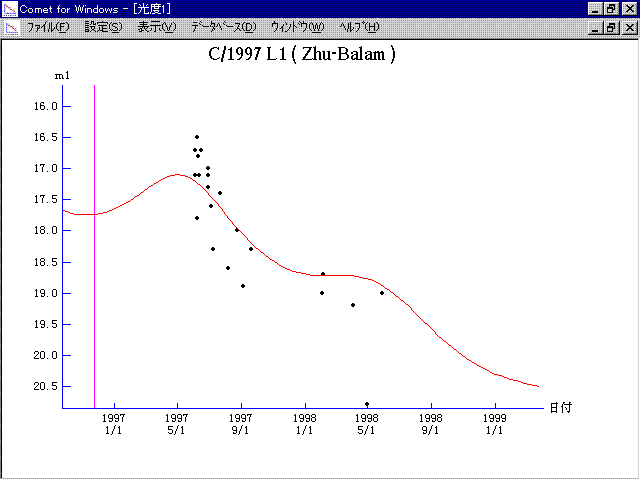# \$B

C/1997 L1 ( Zhu-Balam )###\$B%W%m%U%#!<%k(B

 \$BH/8+F|(B 1997\$BG/(B6\$B7n(B3\$BF|(B \$BH/8+8wEY(B 16.7\$BEy(B \$BH/8+ J. Zhu (Beijing Astronomical Observatory)

###\$B50F;MWAG(B

```   The following improved orbital elements by Kenji Muraoka, are
from 199 observations 1997 June 3 to 1998 Aug. 30, perturbations
by 9 Planets, Moon and 5 minor planets were taken into account.
The mean residual is +/- 0.70 arc seconds.

Epoch  =  1996 Nov. 13.0  TT       JDT = 2450400.5
T  =  1996 Nov. 22.70732       +/- 0.00558 (m.e.) TT
Peri. =  346.34250                +/- 0.00065
Node  =  233.29917                +/- 0.00048   (2000.0)
Incl. =   72.98609                +/- 0.00055
q  =    4.8992595              +/- 0.0000425 AU
e  =    0.9981514              +/- 0.0000194
1/a  =   +0.0003773              +/- 0.0000040 1/AU
orig. 1/a  =   +0.000574  1/AU
fut.  1/a  =   +0.000906  1/AU
```

###\$B@1?^(B1996\$BG/(B 1\$B7n(B 8\$BF|!A(B1998\$BG/(B11\$B7n(B13\$BF|(B

###\$B8wEYJQ2=(B

```        m1 = 7.0 + 5 log\$B&\$(B + 10.0 log r
```##### \$B50F;MWAG\$OB<2,7r<#;a\$N7W;;\$K\$h\$k\$b\$N\$G\$9!#(B \$B@1?^\$O(B StellaNavigator Ver.2.0 for Windows (\$B%"%9%H%m%"!<%D(B \$BJTCx(B / \$B%"%9%-!<=PHG6I4)(B) \$B\$G:n@.\$7\$?\$b\$N\$G\$9!#(B \$B8wEY%0%i%U\$O(BComet for Windows\$B\$G:n@.\$7\$?\$b\$N\$G\$9!#(B